# Example IIR-FIR filter

Submitted by mic on Fri, 03/30/2018 - 10:39

admin: First posted on 2016 07 09

Guest post by Anton Kamenov

The following is an example frequency filter that combines the standard low pass FIR filter with a standard second order low pass Butterworth filter. We are doing this to show that we do not have to stick to the standard filter design, but can experiment. Relying on the standard filters is a start, but there is much we can do.

The standard finite impulse response (FIR) filter has a narrow transition band, but does not have great stop band attenuation. The Butterworth filter – at least the low order Butterworth filters that are easy to put together – has great stop band attenuation, but a wide transition band. It is natural to try and combine the two filters. The most obvious way to do so is to stack the filters one after the other. We can run the signal, for example, first through the Butterworth filter and then through the FIR filter. Alternatively, we can use the fact that the total transfer function of the overall result (of the two stacked filters) is the product of the transfer functions of the individual filters.

A second order low pass Butterworth filter at the cutoff frequency ωc = 0.6 is given by the following transfer function.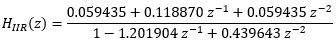A, say, 21 point low pass FIR filter, given the sampling frequency fs = 2000 Hz and with the same cutoff frequency fc = fs ωc / 2π = 190.98 Hz is given by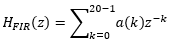where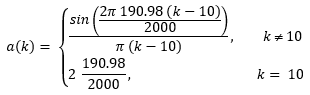The total filter then would have the transfer function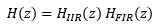This particular filter has the magnitude response shown on the figure below. As expected, this filter has a much narrower transition band than the Butterworth filter and much better stop band attenuation than the FIR filter. These are achieved at the expense of introducing ripples (the Gibbs phenomenon ripples), which the FIR filter naturally produces (we could use windows on FIR filter).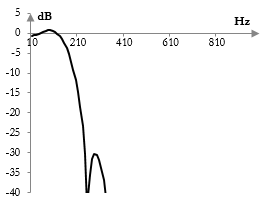What is more interesting is that we can now downsample the FIR filter coefficients. Rather than usingwe can use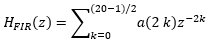This means that we will use every second coefficient of the FIR filter. Normally, in a single low pass filter at fc, downsampling will introduce aliasing (i.e., a "confusion of frequencies"). Using every second coefficient, for example, will produce two pass bands: one between 0 and fc and one between (fs / 2) – fc and (fs / 2). Here, however, we can rely on the fact that the FIR filter is applied together with a Butterworth filter and that the magnitude response of the Butterworth filter is such that the second pass band, between (fs / 2) – fc and (fs / 2), will not be produced.

Although downsampling produces other pass bands due to aliasing, it may have negligible impacts on the original pass band. The following figure, for example, shows the impact on the original pass band of downsampling by a factor of eight (which would normally produce multiple pass bands) of a filter of 101 points at fc = 40 Hz. The dotted line is the magnitude response of the original filter.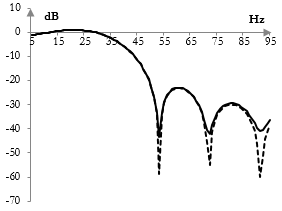If we downsample the FIR filter in our original IIR-FIR filter prototype, we will produce an (almost) identical magnitude response as in the first figure above. This means that we will end up with a total filter of 13 points – 11 in the numerator and 2 in the denominator. This filter is comparable to, say, the fourth order Butterworth filter (9 points), but has a narrower transition band at the expense of ripples in the pass band.

### Filtered HTML

• Freelinking helps you easily create HTML links. Links take the form of [[indicator:target|Title]]. By default (no indicator): Click to view a local node.